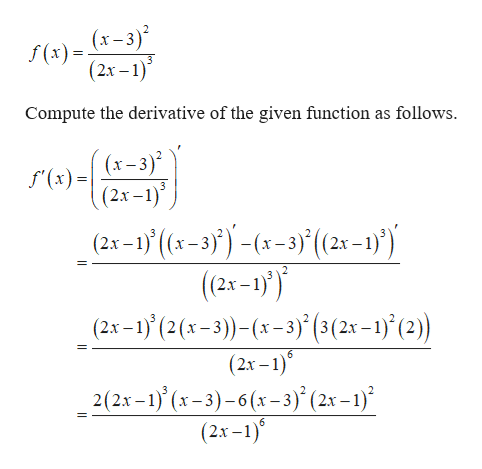# Find the derivative of the function:f(x)=ln  (x-3)^2÷(2x-1)^3

Question
16 views

Find the derivative of the function:

f(x)=ln  (x-3)^2÷(2x-1)^3

check_circle

Step 1

Given function is,&...help_outlineImage Transcriptionclose(х-3)* f(x) (2х - 1)" Compute the derivative of the given function as follows x) = (x-3)2 (2х -1)° f'(x) (2x-1)' (s-3))-(x-3)(2-1) (2x-1)') (2x-1) (2(x-3))-(x-3) (3(2x-1)' (2)) _ (2х - 1)" (2x-1)(x-3)-6(x-3) (2x -1) (2x-1) fullscreen

### Want to see the full answer?

See Solution

#### Want to see this answer and more?

Solutions are written by subject experts who are available 24/7. Questions are typically answered within 1 hour.*

See Solution
*Response times may vary by subject and question.
Tagged in

### Derivative